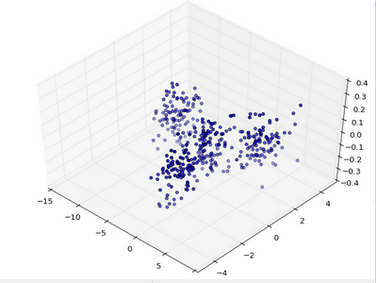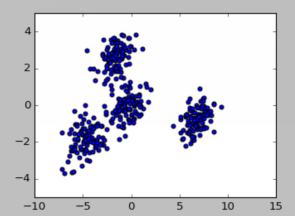写在前面

Principle Component Analysis 顾名思义，是通过分析向量空间的主成分，将主成分提取出来，不重要的成分略去，从而达到降维压缩信息的目的。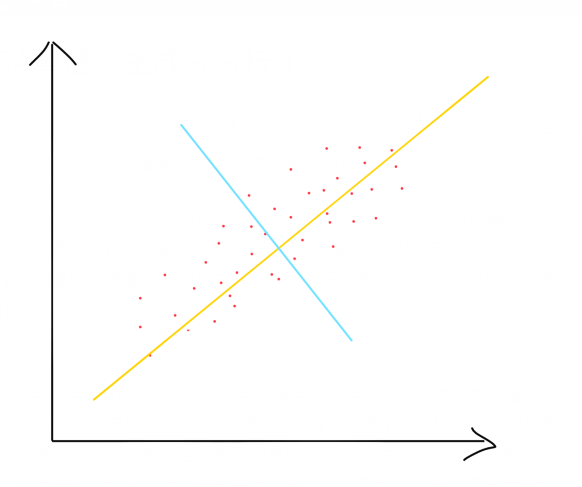投影

 A * B = |A||B|cos(a)


|B|=1时，

 A * B= |A|cos(a)


讨论二维空间基变换

 x = r * cos(θ)   y = r * sin(θ)


 (1,0) 和 (0,1)


x和y相当于投影后的长度。

（注意，新基的坐标是在基本基上确定的）

12121212(32)=5212

(52,12)

多维空间基变换

M个N维向量（组成矩阵A），将其变到R个N维基（组成矩阵B）表示的新空间中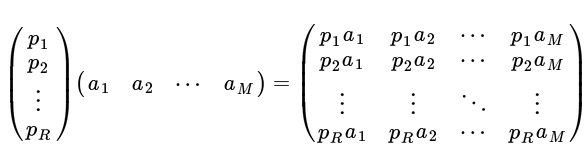协方差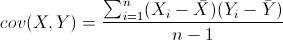• 如果结果为正值，则说明两者是正相关的。
• 如果结果为负值，则说明两者是负相关的。
• 如果为0，也是就是统计上说的“相互独立”。

a,b两个字段组成X，
（相当于m个点的x和y值）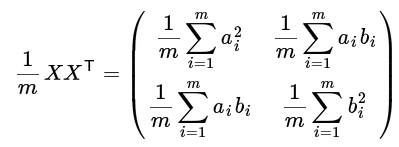PCA计算

X：原始矩阵
C : X对应的协方差矩阵
P : 新基的矩阵
Y = PX
Y : X对P做基变换后的矩阵
D : Y的协方差矩阵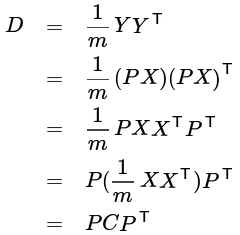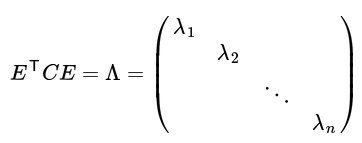（E为正交矩阵）

P=ET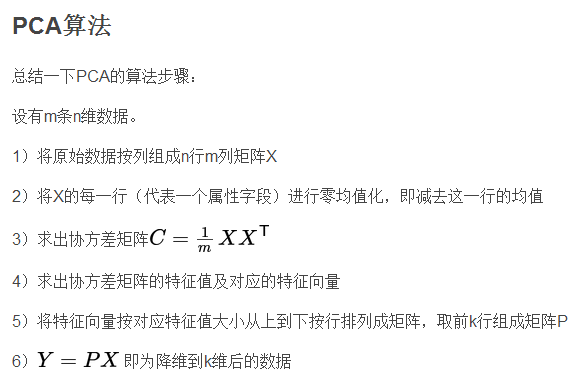程序实现

"""
Author: totodum
Program: Linear_PCA.py
Description: Linear Principle Component Analysis
"""
from numpy import *
from numpy.linalg import *

'''
PCA takes an array of points, each row is a single point. and reduce the dimension
of the points to k-D.
return points of k-D.
'''

def pca(x, k):
m = x.shape
c = dot(transpose(x), x) / m  # coefficient
[u, s, v] = svd(c)
p = u[:, 0:k]
y = dot(x, p)
return y